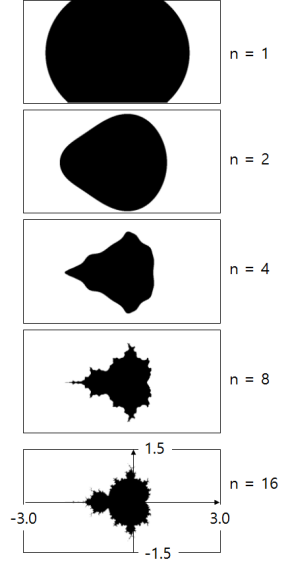# Mandelbrot Set

• This simulation supports both pinch zoom and mouse scrolling.
• We recommend using the Chrome browser. (to get more processing speed)

Imaginary number

An imaginary number is consisted with a number that become negative if you square itself.
An imaginary number is represented by the letter ‘i’. This means that the imaginary number doesn’t actually exist.

i2 = -1

Imaginary numbers are a whole new number system different from real numbers. This is because it become positive numbers when you square real number.
Imaginary numbers are not numbers that exists in the real world. But, it comes with logical error free and can be calculated using a computer.
You can indicate the imaginary scale by prefixing the imaginary numbers with real numbers. (ex. i, 2i, 3i, 1.5i). But you can’t compare the magnitude of an imaginary number. That is, 2i is not bigger than i. This is because imaginary numbers are neither positive nor negative.

(bi)2 = b2i2 = -b2

Complex number

A complex number is a number like ‘a + bi’ that mixes real number and imaginary number.
If ‘b = 0’ in the complex ‘a + bi’, only the real part remains.
If ‘a = 0’ in the complex ‘a + bi’, the real part disappears and only the pure imaginary ‘bi’ remains.

Representation of a complex number

Complex numbers can be represented in the plane. In other words, the x-axis is the axis that represents the real number, and the y-axis that is perpendicular to the x-axis represents the imaginary number.
Pure real numbers then correspond to the horizontal axis, pure imaginary numbers correspond to the vertical axis. And complex number correspond to one point on the plane not the axis.Mandelbrot Set

Let’s learn about the Mandelbrot equation. Mandelbro’s equation is a very simple equation with only two variables, ‘Z’ and ‘C’.

Zn+1 = Zn2 + C

Square the complex number ‘Z’ and add ‘C’ to it to create a new ‘Z’. Square the newly created ‘Z’ and then add ‘C’ to it again to create a new ‘Z’. Repeat this infinitely.

Mandelbrot found that the value of ‘Z’ continued to increase or to shuttle between two very small imaginary numbers (depending on the value of ‘C’). He used a computer to represent each ‘C’ value on the screen as a point on which ‘Z’ does not radiate. The result is something far from purely geometric shapes (squares, triangles, circles). This is called Mandelbrot’s set.
Mandelbrot continued to magnify the image, but the same structure continued to appear. Even after zooming in forever, the same shape and structure continued to emerge.How to draw Mandelbrot’s set

First, set a complex coordinate (x, y) on one pixel of the computer screen and call it C (= x, yi). Normally, the x value ranges from -3 to +3, and the y value ranges from -2 to +2.
With this range, you can see the whole view of Mandelbrot’s set.Then, if the values ​​that Z does not diverge represent as black dots on the screen, the result is a Mandelbrot set. Normally, if it is larger than 2 ~ 4, it is regarded as diverging and stops the recurrence relation.The more you repeat the recurrence relation, the finer the picture.
However, due to the limitations of computer computing power, it cannot be calculated indefinitely, so it stops at a reasonable level.

You can get an artistic picture if an appropriate color is applied to the screen according to the Iteration number of the moment when the divergence is determined. Currently, Mandelbrot’s set becomes the main material for graphics that utilizes computer’s computing power along with Julia’s set.